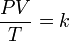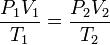# Combined gas law

The combined gas law which combines Charles's law, Boyle's law, and Gay-Lussac's law. These laws each relate one thermodynamic variable to another mathematically while holding everything else constant. Charles's law states that volume and temperature of an ideal gas are directly proportional to each other as long as everything is held constant. Boyle's law asserts that pressure and volume of an ideal gas are inversely proportional to each other at fixed temperature. Finally, Gay-Lussac's law introduces a direct proportionality between temperature and pressure of an ideal gas as long as it is at a constant volume. The inter-dependence of these variables is shown in the combined gas law, which clearly states that:

The ratio between the pressure-volume constant and the temperature of an ideal gas remains constant.

This can be stated mathematically as$\qquad \frac {PV}{T}= k$

where:

P is the pressure,
V is the volume,
T is the absolute temperature,
k is a constant (with units of energy divided by temperature).

For comparing the same substance under two different sets of conditions, the law can be written as:$\qquad \frac {P_1V_1}{T_1}= \frac {P_2V_2}{T_2}$

The addition of Avogadro's law to the combined gas law yields the ideal gas law.Some content on this page may previously have appeared on Citizendium.